# Resources tagged with: Coordinates

Filter by: Content type:
Age range:
Challenge level:

### There are 23 results

Broad Topics > Coordinates, Functions and Graphs > Coordinates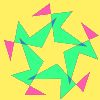### Attractive Rotations

##### Age 11 to 14 Challenge Level:

Here is a chance to create some attractive images by rotating shapes through multiples of 90 degrees, or 30 degrees, or 72 degrees or...### Parallel Parking

##### Age 14 to 16

Scientist Bryan Rickett has a vision of the future - and it is one in which self-parking cars prowl the tarmac plains, hunting down suitable parking spots and manoeuvring elegantly into them.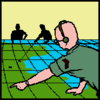### Going Places with Mathematicians

##### Age 7 to 14

This article looks at the importance in mathematics of representing places and spaces mathematics. Many famous mathematicians have spent time working on problems that involve moving and mapping. . . .### Corridors

##### Age 14 to 16 Challenge Level:

A 10x10x10 cube is made from 27 2x2 cubes with corridors between them. Find the shortest route from one corner to the opposite corner.### Hypotenuse Lattice Points

##### Age 14 to 16 Challenge Level:

The triangle OMN has vertices on the axes with whole number co-ordinates. How many points with whole number coordinates are there on the hypotenuse MN?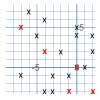### Ten Hidden Squares

##### Age 7 to 14 Challenge Level:

These points all mark the vertices (corners) of ten hidden squares. Can you find the 10 hidden squares?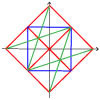### Coordinate Designs

##### Age 11 to 14 Challenge Level:### Coordinate Patterns

##### Age 11 to 14 Challenge Level:

Charlie and Alison have been drawing patterns on coordinate grids. Can you picture where the patterns lead?### Square Coordinates

##### Age 11 to 14 Challenge Level:

A tilted square is a square with no horizontal sides. Can you devise a general instruction for the construction of a square when you are given just one of its sides?### Route to Infinity

##### Age 11 to 14 Challenge Level:

Can you describe this route to infinity? Where will the arrows take you next?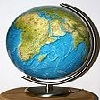### Coordinates and Descartes

##### Age 7 to 16

Have you ever wondered how maps are made? Or perhaps who first thought of the idea of designing maps? We're here to answer these questions for you.### Something in Common

##### Age 14 to 16 Challenge Level:

A square of area 3 square units cannot be drawn on a 2D grid so that each of its vertices have integer coordinates, but can it be drawn on a 3D grid? Investigate squares that can be drawn.### 3D Treasure Hunt

##### Age 14 to 18 Challenge Level:

Some treasure has been hidden in a three-dimensional grid! Can you work out a strategy to find it as efficiently as possible?### Hidden Squares

##### Age 11 to 14 Challenge Level:

Can you find the squares hidden on these coordinate grids?### Treasure Hunt

##### Age 7 to 14 Challenge Level:

Can you find a reliable strategy for choosing coordinates that will locate the treasure in the minimum number of guesses?### Isosceles Triangles

##### Age 11 to 14 Challenge Level:

Draw some isosceles triangles with an area of $9$cm$^2$ and a vertex at (20,20). If all the vertices must have whole number coordinates, how many is it possible to draw?### A Tilted Square

##### Age 14 to 16 Challenge Level:

The opposite vertices of a square have coordinates (a,b) and (c,d). What are the coordinates of the other vertices?### Beelines

##### Age 14 to 16 Challenge Level:

Is there a relationship between the coordinates of the endpoints of a line and the number of grid squares it crosses?### Eight Hidden Squares

##### Age 7 to 14 Challenge Level:

On the graph there are 28 marked points. These points all mark the vertices (corners) of eight hidden squares. Can you find the eight hidden squares?##### Age 14 to 16 Challenge Level:

A 1 metre cube has one face on the ground and one face against a wall. A 4 metre ladder leans against the wall and just touches the cube. How high is the top of the ladder above the ground?### Just Opposite

##### Age 14 to 16 Challenge Level:

A and C are the opposite vertices of a square ABCD, and have coordinates (a,b) and (c,d), respectively. What are the coordinates of the vertices B and D? What is the area of the square?